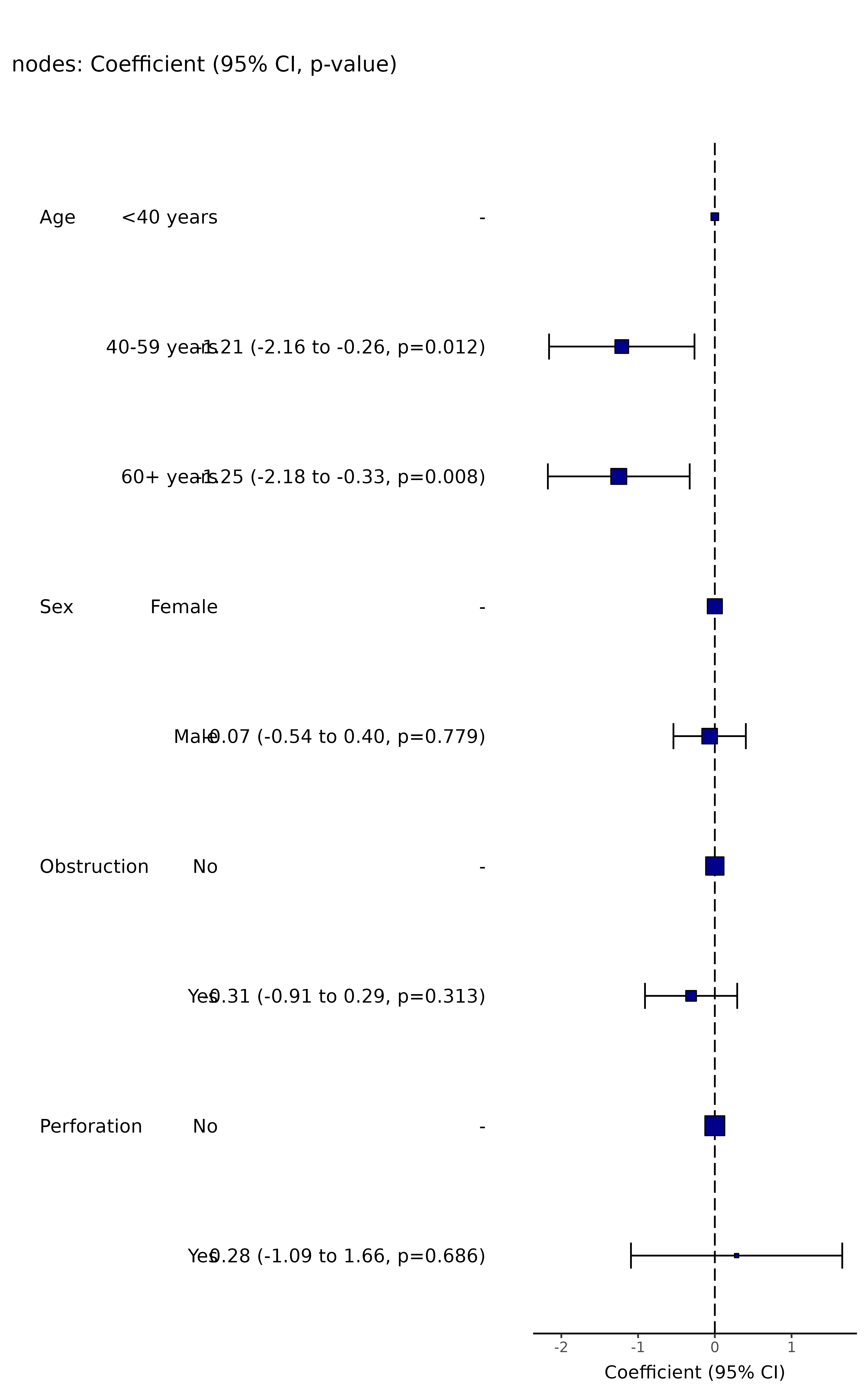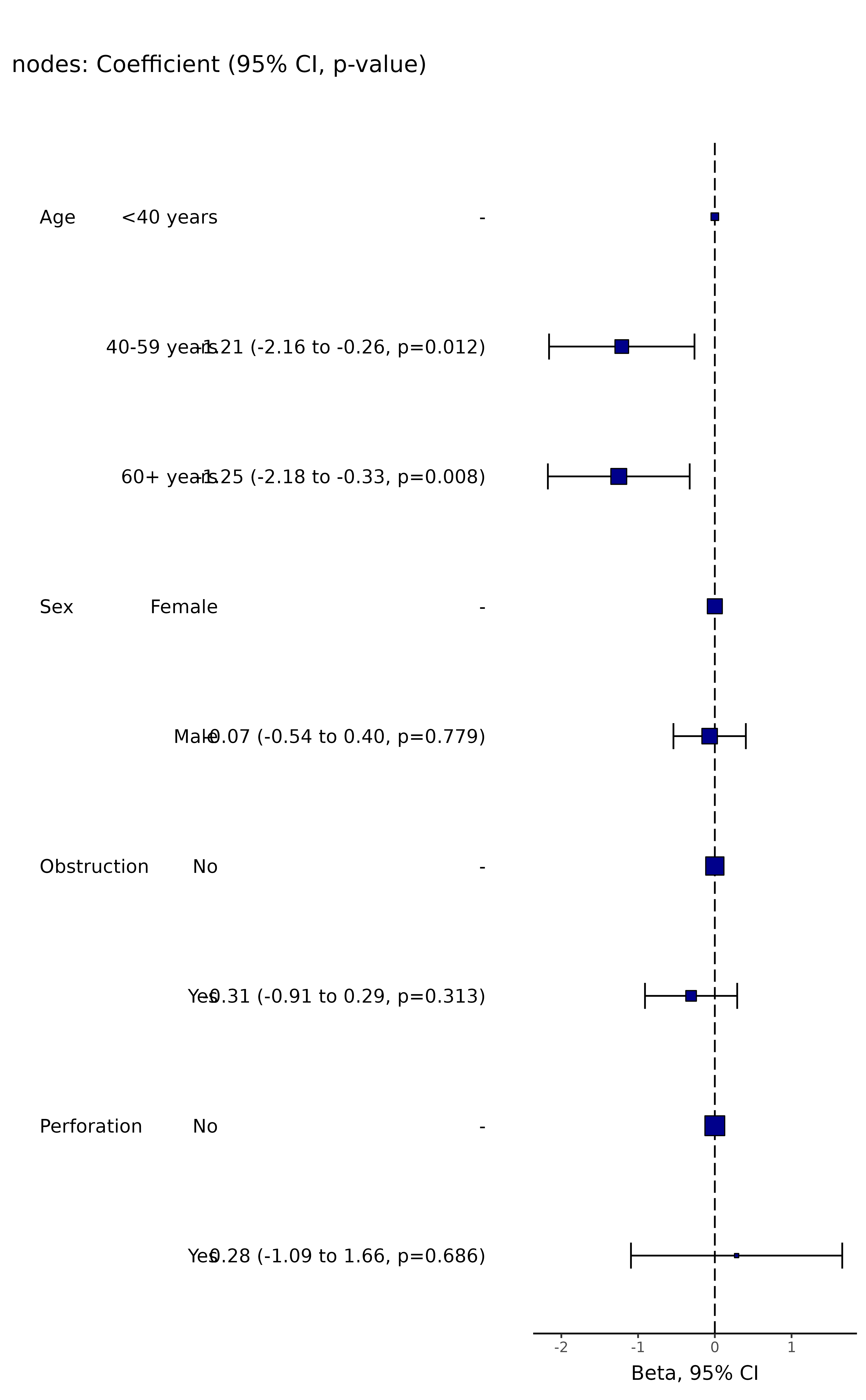Produce a coefficient and plot from a lm() model.

coefficient_plot(
.data,
dependent,
explanatory,
random_effect = NULL,
factorlist = NULL,
lmfit = NULL,
confint_type = "default",
remove_ref = FALSE,
breaks = NULL,
column_space = c(-0.5, -0.1, 0.5),
dependent_label = NULL,
prefix = "",
suffix = ": Coefficient, 95% CI, p-value)",
table_text_size = 5,
title_text_size = 18,
plot_opts = NULL,
table_opts = NULL,
...
)

## Arguments

.data

Dataframe.

dependent

Character vector of length 1: name of depdendent variable (must be numeric/continuous).

explanatory

Character vector of any length: name(s) of explanatory variables.

random_effect

Character vector of length 1, name of random effect variable.

factorlist

Option to provide output directly from summary_factorlist().

lmfit

Option to provide output directly from lmmulti() and lmmixed().

confint_type

For for lmer models, one of c("default", "Wald", "profile", "boot") Note "default" == "Wald".

remove_ref

Logical. Remove reference level for factors.

breaks

Manually specify x-axis breaks in format c(0.1, 1, 10).

column_space

Adjust table column spacing.

dependent_label

Main label for plot.

prefix

Plots are titled by default with the dependent variable. This adds text before that label.

suffix

Plots are titled with the dependent variable. This adds text after that label.

table_text_size

Alter font size of table text.

title_text_size

Alter font size of title text.

plot_opts

A list of arguments to be appended to the ggplot call by "+".

table_opts

A list of arguments to be appended to the ggplot table call by "+".

...

Other parameters.

## Value

Returns a table and plot produced in ggplot2.

Other finalfit plot functions: ff_plot(), hr_plot(), or_plot(), surv_plot()

## Examples

library(finalfit)
library(ggplot2)

# Coefficient plot
explanatory = c("age.factor", "sex.factor", "obstruct.factor", "perfor.factor")
dependent = "nodes"
colon_s %>%
coefficient_plot(dependent, explanatory)
#> Note: dependent includes missing data. These are dropped.
#> Warning: Removed 4 rows containing missing values (geom_errorbarh).colon_s %>%
coefficient_plot(dependent, explanatory, table_text_size=4, title_text_size=14,
plot_opts=list(xlab("Beta, 95% CI"), theme(axis.title = element_text(size=12))))
#> Note: dependent includes missing data. These are dropped.
#> Warning: Removed 4 rows containing missing values (geom_errorbarh).